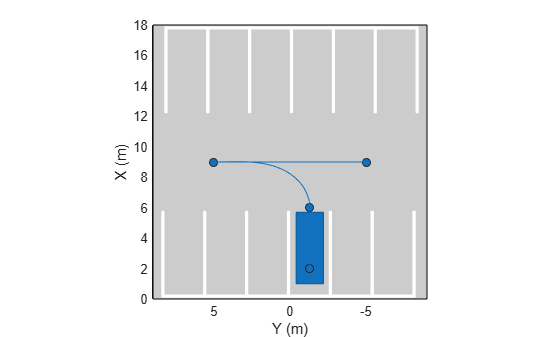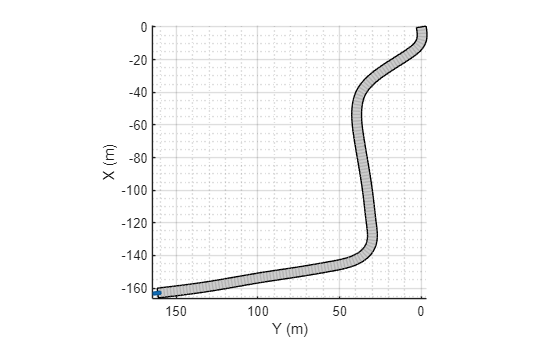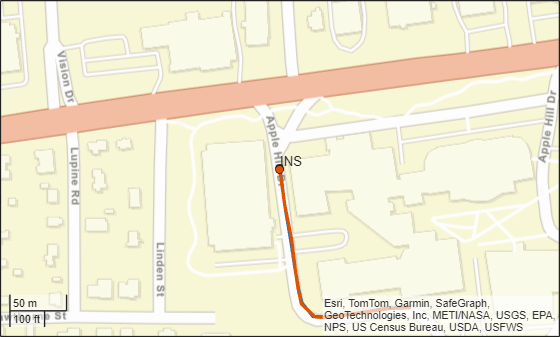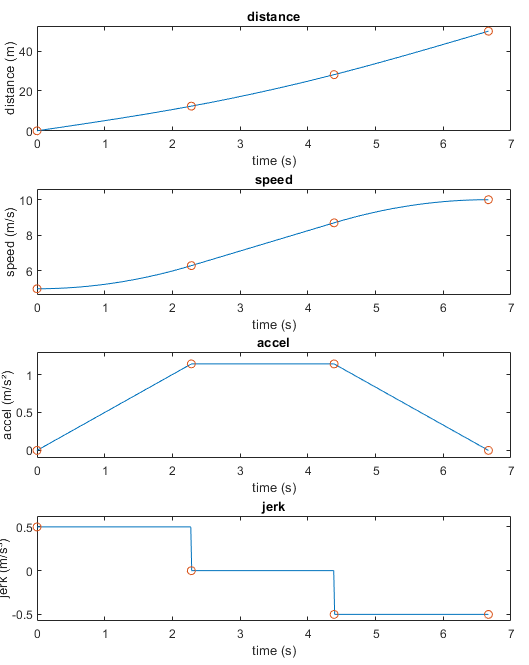# smoothTrajectory

Create smooth, jerk-limited actor trajectory in driving scenario

Since R2021a

## Syntax

``smoothTrajectory(ac,waypoints)``
``smoothTrajectory(ac,waypoints,speed)``
``smoothTrajectory(ac,waypoints,speed,waittime)``
``smoothTrajectory(___,Name,Value)``

## Description

The `smoothTrajectory` function creates a smooth, jerk-limited trajectory for an actor in a driving scenario. The generated trajectory features a smooth transition of accelerations between waypoints, making it compatible for generating synthetic inertial navigation system (INS) and global navigation satellite system (GNSS) measurements from an `insSensor` System object™. For more details on how `smoothTrajectory` generates trajectories, see Algorithms.

````smoothTrajectory(ac,waypoints)` creates a smooth trajectory for an actor or vehicle, `ac`, to follow from a set of waypoints. The actor travels at a constant speed of 30 meters per second.```

example

````smoothTrajectory(ac,waypoints,speed)` also specifies the speed at which the actor or vehicle travels along the trajectory, in either forward or reverse motion.```

example

````smoothTrajectory(ac,waypoints,speed,waittime)` also specifies wait times for an actor or vehicle. Use this syntax to pause the actor or vehicle at specific waypoints.```

example

````smoothTrajectory(___,Name,Value)` specifies options using one or more name-value pairs and any of the input argument combinations from previous syntaxes. For example, you can specify the yaw orientation angle of the actor or vehicle at each waypoint or the maximum amount of jerk in the trajectory.```

## Examples

collapse all

Create a driving scenario containing a curved two-lane road.

```scenario = drivingScenario('SampleTime',0.05); roadcenters = [0 0; 24.2 27.7; 50 30]; lspec = lanespec(2); road(scenario,roadcenters,'Lanes',lspec); ```

Add a vehicle to the scenario. Set a trajectory in which the vehicle slows down as it enters the curve.

```v = vehicle(scenario,'ClassID',1); waypoints = [2.6 1.0; 23.6 24.9; 45.5 28.6]; speed = [9 8 9]; smoothTrajectory(v,waypoints,speed) ```

Plot the scenario and run the simulation.

```plot(scenario,'Waypoints','on','RoadCenters','on') while advance(scenario) pause(scenario.SampleTime) end ```Create a driving scenario containing a four-way intersection.

```scenario = drivingScenario('SampleTime',0.02,'StopTime',20); roadCenters = [0 0; 50 0]; laneSpecification = lanespec([1 1]); road(scenario,roadCenters,'Lanes',laneSpecification); roadCenters = [25 25; 25 -25]; road(scenario,roadCenters,'Lanes',laneSpecification); ```

Add the ego vehicle, which travels north but waits for one second at the intersection.

```ego = vehicle(scenario,'ClassID',1,'Position',[2 -2 0]); waypoints = [2 -2; 17.5 -2; 45 -2]; speed = [5 0 5]; waittime = [0 1 0]; smoothTrajectory(ego,waypoints,speed,waittime); ```

Add a bicyclist that travels east through the intersection at a constant speed without stopping.

```bicycle = actor(scenario, ... 'ClassID',3, ... 'Length',1.7, ... 'Width',0.45, ... 'Height',1.7, ... 'Position',[23 23 0]); waypoints = [23 23; 23 -23]; speed = 4; smoothTrajectory(bicycle,waypoints,speed); ```

Plot the scenario. The vehicle stops at the intersection for one second, then resumes driving after the bicyclist crosses the intersection.

```plot(scenario) while advance(scenario) pause(scenario.SampleTime) end ```Simulate a driving scenario in which a car drives in reverse to back into a parking space.

Create a driving scenario containing a parking lot.

```scenario = drivingScenario; vertices = [0 9; 18 9; 18 -9; 0 -9]; parkingLot(scenario,vertices,ParkingSpace=parkingSpace); ```

Create a car and define its trajectory. The car drives forward, stops, and then drives in reverse to back into the parking space. As the car enters the parking space, it has a yaw orientation angle that is 90 degrees counterclockwise from where it started.

```car = vehicle(scenario,ClassID=1); waypoints = [9 -5; 9 5; 6 -1.3; 2 -1.3]; speed = [3; 0; -2; 0]; yaw = [90 90 180 180]; smoothTrajectory(car,waypoints,speed,Yaw=yaw) ```

Plot the driving scenario and display the waypoints of the trajectory.

```plot(scenario,Waypoints="on") while advance(scenario) pause(0.001) end ```Create the trajectory of a pedestrian who takes a sharp right turn at an intersection.

```scenario = drivingScenario; roadCenters = [0 10; 0 -10]; road(scenario,roadCenters); road(scenario,flip(roadCenters,2)); ```

Add a pedestrian actor to the scenario.

```pedestrian = actor(scenario, ... 'ClassID',4, ... 'Length',0.24, ... 'Width',0.45, ... 'Height',1.7, ... 'Position',[-9 0 0], ... 'RCSPattern',[-8 -8; -8 -8], ... 'Mesh',driving.scenario.pedestrianMesh, ... 'Name','Pedestrian'); ```

Define the trajectory of the pedestrian. The pedestrian approaches the intersection, pauses briefly, and then takes a sharp right turn at the intersection. To define the sharp right turn, specify two waypoints at the intersection that are close together. For these waypoints, specify the yaw orientation angle of the second waypoint at a 90-degree angle from the first waypoint.

```waypoints = [-9 0; -0.25 0; 0 -0.25; 0 -9]; speed = [1.5; 0; 0.5; 1.5]; yaw = [0; 0; -90; -90]; waittime = [0; 0.2; 0; 0]; smoothTrajectory(pedestrian,waypoints,speed,waittime,'Yaw',yaw); ```

Plot the driving scenario and display the waypoints of the pedestrian.

```plot(scenario,'Waypoints','on') while advance(scenario) pause(0.001) end ```Generate measurements from an INS sensor that is mounted to a vehicle in a driving scenario. Plot the INS measurements against the ground truth state of the vehicle and visualize the velocity and acceleration profile of the vehicle.

Create Driving Scenario

Load the geographic data for a driving route at the MathWorks® Apple Hill campus in Natick, MA.

```data = load('ahroute.mat'); latIn = data.latitude; lonIn = data.longitude; ```

Convert the latitude and longitude coordinates of the route to Cartesian coordinates. Set the origin to the first coordinate in the driving route. For simplicity, assume an altitude of 0 for the route.

```alt = 0; origin = [latIn(1),lonIn(1),alt]; [xEast,yNorth,zUp] = latlon2local(latIn,lonIn,alt,origin); ```

Create a driving scenario. Set the origin of the converted route as the geographic reference point.

```scenario = drivingScenario('GeoReference',origin); ```

Create a road based on the Cartesian coordinates of the route.

```roadCenters = [xEast,yNorth,zUp]; road(scenario,roadCenters); ```

Create a vehicle that follows the center line of the road. The vehicle travels between 4 and 5 meters per second (9 to 11 miles per hour), slowing down at the curves in the road. To create the trajectory, use the `smoothTrajectory` function. The computed trajectory minimizes jerk and avoids discontinuities in acceleration, which is a requirement for modeling INS sensors.

```egoVehicle = vehicle(scenario,'ClassID',1); egoPath = roadCenters; egoSpeed = [5 5 5 4 4 4 5 4 4 4 4 5 5 5 5 5]; smoothTrajectory(egoVehicle,egoPath,egoSpeed); ```

Plot the scenario and show a 3-D view from behind the ego vehicle.

```plot(scenario) chasePlot(egoVehicle) ```Create INS Sensor

Create an INS sensor that accepts the input of simulation times. Introduce noise into the sensor measurements by setting the standard deviation of velocity and accuracy measurements to 0.1 and 0.05, respectively.

```INS = insSensor('TimeInput',true, ... 'VelocityAccuracy',0.1, ... 'AccelerationAccuracy',0.05); ```

Visualize INS Measurements

Initialize a geographic player for displaying the INS measurements and the actor ground truth. Configure the player to display its last 10 positions and set the zoom level to 17.

```zoomLevel = 17; player = geoplayer(latIn(1),lonIn(1),zoomLevel, ... 'HistoryDepth',10,'HistoryStyle','line'); ```Pre-allocate space for the simulation times, velocity measurements, and acceleration measurements that are captured during simulation.

```numWaypoints = length(latIn); times = zeros(numWaypoints,1); gTruthVelocities = zeros(numWaypoints,1); gTruthAccelerations = zeros(numWaypoints,1); sensorVelocities = zeros(numWaypoints,1); sensorAccelerations = zeros(numWaypoints,1); ```

Simulate the scenario. During the simulation loop, obtain the ground truth state of the ego vehicle and an INS measurement of that state. Convert these readings to geographic coordinates, and at each waypoint, visualize the ground truth and INS readings on the geographic player. Also capture the velocity and acceleration data for plotting the velocity and acceleration profiles.

```nextWaypoint = 2; while advance(scenario) % Obtain ground truth state of ego vehicle. gTruth = state(egoVehicle); % Obtain INS sensor measurement. measurement = INS(gTruth,scenario.SimulationTime); % Convert readings to geographic coordinates. [latOut,lonOut] = local2latlon(measurement.Position(1), ... measurement.Position(2), ... measurement.Position(3),origin); % Plot differences between ground truth locations and locations reported by sensor. reachedWaypoint = sum(abs(roadCenters(nextWaypoint,:) - gTruth.Position)) < 1; if reachedWaypoint plotPosition(player,latIn(nextWaypoint),lonIn(nextWaypoint),'TrackID',1) plotPosition(player,latOut,lonOut,'TrackID',2,'Label','INS') % Capture simulation times, velocities, and accelerations. times(nextWaypoint,1) = scenario.SimulationTime; gTruthVelocities(nextWaypoint,1) = gTruth.Velocity(2); gTruthAccelerations(nextWaypoint,1) = gTruth.Acceleration(2); sensorVelocities(nextWaypoint,1) = measurement.Velocity(2); sensorAccelerations(nextWaypoint,1) = measurement.Acceleration(2); nextWaypoint = nextWaypoint + 1; end if nextWaypoint > numWaypoints break end end ```Plot Velocity Profile

Compare the ground truth longitudinal velocity of the vehicle over time against the velocity measurements captured by the INS sensor.

Remove zeros from the time vector and velocity vectors.

```times(times == 0) = []; gTruthVelocities(gTruthVelocities == 0) = []; sensorVelocities(sensorVelocities == 0) = []; figure hold on plot(times,gTruthVelocities) plot(times,sensorVelocities) title('Longitudinal Velocity Profile') xlabel('Time (s)') ylabel('Velocity (m/s)') legend('Ground truth','INS') hold off ```Plot Acceleration Profile

Compare the ground truth longitudinal acceleration of the vehicle over time against the acceleration measurements captured by the INS sensor.

```gTruthAccelerations(gTruthAccelerations == 0) = []; sensorAccelerations(sensorAccelerations == 0) = []; figure hold on plot(times,gTruthAccelerations) plot(times,sensorAccelerations) title('Longitudinal Acceleration Profile') xlabel('Time (s)') ylabel('Acceleration (m/s^2)') legend('Ground truth','INS') hold off ```## Input Arguments

collapse all

Actor belonging to a `drivingScenario` object, specified as an `Actor` or `Vehicle` object. To create these objects, use the `actor` and `vehicle` functions, respectively.

Trajectory waypoints, in meters, specified as a real-valued N-by-2 or N-by-3 matrix. N is the number of waypoints.

• If `waypoints` is an N-by-2 matrix, then each matrix row represents the (x, y) coordinates of a waypoint. The z-coordinate of each waypoint is zero.

• If `waypoints` is an N-by-3 matrix, then each matrix row represents the (x, y, z) coordinates of a waypoint.

Each of the N – 1 segments between the waypoints defines a curve whose curvature varies linearly with length. If the first and last waypoint are identical, then the trajectory forms a loop.

Waypoints are in the world coordinate system.

Example: `[1 0 0; 2 7 7; 3 8 8]`

Data Types: `single` | `double`

Speed of the actor at each waypoint, in meters per second, specified as a real-valued scalar or N-element real-valued vector. N is the number of waypoints specified by `waypoints`.

• When `speed` is a scalar, the speed is constant throughout the actor motion.

• When `speed` is a vector, the vector values specify the speed at each waypoint. For forward motion, specify positive speed values. For reverse motion, specify negative speed values. To change motion directions, separate the positive and negative speeds by a waypoint with `0` speed.

Speeds are interpolated between waypoints. You can specify `speed` values as `0` at any waypoint but you cannot specify `0` speed at two consecutive waypoints.

If you do not specify `speed`, then by default, the actor travels at a constant speed of 30 m/s.

Example: `[10 8 9]` specifies speeds of 10 m/s, 8 m/s, and 9 m/s.

Example: `[10 0 -10]` specifies a speed of 10 m/s in forward motion, a pause for changing directions, and a speed of 10 m/s in reverse.

Data Types: `single` | `double`

Wait time of the actor at each waypoint, in seconds, specified as an N-element real-valued vector. N is the number of waypoints specified by `waypoints`.

When you specify a nonnegative wait time for the actor at a waypoint, the actor pauses at that waypoint for the specified number of seconds. When you specify a nonnegative wait time, you must set the corresponding `speed` value to `0`. You can set the `waitime` to `0` at any waypoint, but you cannot set `waittime` at two consecutive waypoints to nonzero values.

Example: `[0 0 5 0]` pauses the actor for five seconds when it reaches the third waypoint.

Data Types: `single` | `double`

### Name-Value Arguments

Specify optional pairs of arguments as `Name1=Value1,...,NameN=ValueN`, where `Name` is the argument name and `Value` is the corresponding value. Name-value arguments must appear after other arguments, but the order of the pairs does not matter.

Before R2021a, use commas to separate each name and value, and enclose `Name` in quotes.

Example: `'Yaw',[0 90 90 0],'Jerk',0.9`

Yaw orientation angle of the actor at each waypoint, in degrees, specified as the comma-separated pair consisting of `'Yaw'` and an N-element real-valued vector. N is the number of waypoints specified by `waypoints`. Angles are positive in the counterclockwise direction.

If you do not specify `yaw`, then the yaw at each waypoint is `NaN`, meaning that the yaw has no constraints.

Example: `[0 90]` specifies an actor at a 0-degree angle at the first waypoint and a 90-degree angle at the second waypoint.

Example: `[0 NaN]` specifies an actor at a 0-degree angle at the first waypoint. The actor has no constraints on its yaw at the second waypoint.

Data Types: `single` | `double`

Maximum longitudinal jerk of the actor, in meters per second cubed, specified as the comma-separated pair consisting of `'Jerk'` and a real-valued scalar greater than or equal to 0.1.

To limit jerk to a range that creates comfortable trajectories for human passengers, set `'Jerk'` in the range from `0.3` to `0.9` .

Data Types: `single` | `double`

## Tips

• If the `smoothTrajectory` function is unable to compute a smooth, jerk-limited trajectory given the input parameters, try making these adjustments to the scenario:

• Extend the distances between waypoints to give the vehicle more time to accelerate to the specified speeds.

• Lower the speeds at each waypoint. Try converting the speed values from meters per second to miles per hour to see if the speeds are realistic given the scenario. For example, it is unlikely that the algorithm can compute a smooth trajectory for a sharp turn that is taken at a speed of 30 m/s (about 67 mph).

• Increase the maximum jerk. Increasing the jerk maximum enables the algorithm to compute more possible trajectories at the expense of reduced human passenger comfort.

## Algorithms

The `smoothTrajectory` function creates a jerk-limited trajectory using a trapezoidal acceleration profile. This trajectory has smooth acceleration transitions between waypoints, resulting in a comfortable ride for human passengers. The function calculates a separate trapezoidal acceleration profile for each of the N - 1 segments between trajectory waypoints.

Consider a simple scenario in which a car travels a distance of 50 meters along a 100-meter road. The trajectory consists of one 50-meter segment in which the car must increase its speed from 5 m/s to 10 m/s by the end of the segment. The trajectory has an additional constraint in which the maximum longitudinal jerk must not exceed 0.5 m/s3.

```scenario = drivingScenario; car = vehicle(scenario); road(scenario,[0 -25; 0 75]); % m waypoints = [0 0; 0 50]; % m speed = [5 10]; % m/s jerk = 0.5; % m/s^3 smoothTrajectory(car,waypoints,speed,'Jerk',jerk) ```

Given the distance, speed, and jerk constraints of this waypoint segment, the `smoothTrajectory` function generates a three-phase trapezoidal acceleration profile:

1. Increase acceleration linearly. Hold jerk constant at a value no greater than `jerk`.

2. Hold acceleration constant. Decrease jerk to 0.

3. Decrease acceleration linearly. Hold jerk constant at a value no less than `-jerk`.

These plots visualize the distance, speed, acceleration, and jerk profile along this waypoint segment over time. The three phases of the acceleration profile form a trapezoidal shape.When speed decreases between waypoints, the `smoothTrajectory` function generates the three-phase trapezoidal acceleration profile in reverse order. In the decreased speed case, the shape of the acceleration profile is the inverse of the one shown in the previous plot.

 Bae, Il, Jaeyoung Moon, and Jeongseok Seo. "Toward a Comfortable Driving Experience for a Self-Driving Shuttle Bus." Electronics 8, no. 9 (August 27, 2019): 943. https://doi.org/10.3390/electronics8090943.